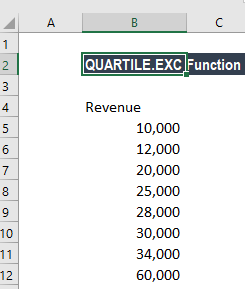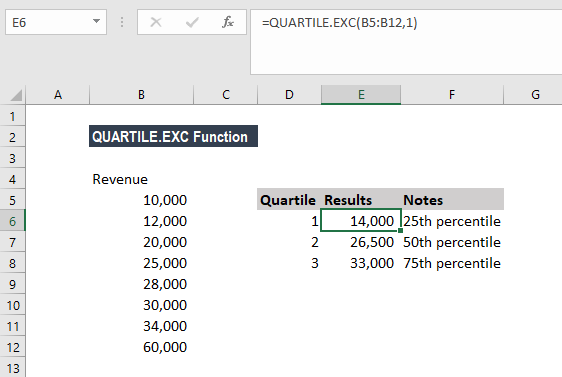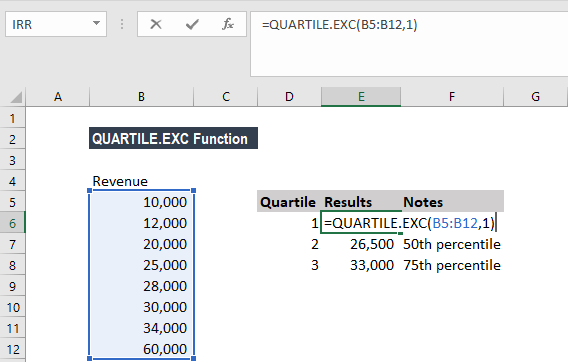# QUARTILE.EXC Function

Returns the quartile of a given data set based on percentile values from 0.1 exclusive

## What is the QUARTILE.EXC Function?

The QUARTILE.EXC Function is categorized under Excel Statistical functions. It will return the quartile of a given data set based on percentile values from 0.1, exclusive. The function bases its calculation on a percentile range of 0 to 1, exclusive.

As a financial analyst, QUARTILE.EXC can be used to find out a specific percentage of incomes in a population. The function is also useful in revenue analysis.

### Formula

=QUARTILE.EXC(array,quart)

The QUARTILE.EXC function uses the following arguments:

1. Array (required argument) – This is the array or cell range of data values for which we want the quartile value.
2. Quart (required argument) – It indicates which value will be returned. It is an integer between 1 and 3, representing the required quartile. If quart = 1 or 3, the supplied array must contain more than 2 values. It accepts three values for the quart argument: 1 (first quartile – 25th percentile), 2 (median value – 50th percentile), and 3 (third quartile – 75th percentile).

### How to use the QUARTILE.EXC Function in Excel?

To understand the uses of the QUARTILE.EXC function, let’s consider an example:

#### Example 1

Suppose we are given the revenue figures below:QUARTILE.EXC can return the first quartile, second quartile, and third quartile, as shown below:The formula used was:Similarly, we could use 2 for the 50th percentile and 3 for the 75th percentile.

### Few notes about the QUARTILE.EXC Function

1. #NUM! error – Occurs if either:
• The given value of quart is < 1 or > 3; or
• The given array is empty; or
• The given array contains less than 3 values and the quart argument is equal to 1 or 3.
2. #VALUE! error – Occurs if the given value of quart is non-numeric.
3. From Excel 2010, the QUARTILE.EXC and QUARTILE.INC functions would replace the QUARTILE function, which is now classified as a compatibility function.
4. The QUARTILE.EXC function cannot calculate the 0’th or the 4’th quartile (as the calculation is based on the range 0 to 1, exclusive).
5. If the requested quartile falls between two of the values in the supplied array, MS Excel interpolates between these values to calculate the quartile value.

Thanks for reading CFI’s guide to the Excel QUARTILE.EXC function. By taking the time to learn and master these Excel functions, you’ll significantly speed up your financial analysis. To learn more, check out these additional CFI resources:

• Excel Functions for Finance